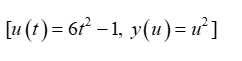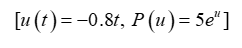# Use the variable u for the inside function to express each of the following as a composite function. Enclose arguments of functions in parentheses. For example, sin(2x). (a) y=(6t2−1)2 Enter your answer as a list with square brackets and terms separated by commas [u(t), y(u)], where u(t) is the inside function and y(u) is the outside function.(b) P=5e−0.8t Enter your answer as a list with square brackets and terms separated by commas [u(t), P(u)], where u(t) is the inside function and P(u) is the outside function.(c) C=4ln(q2+4) Enter your answer as a list with square brackets and terms separated by commas [u(q), C(u)], where u(q) is the inside function and C(u) is the outside function.

Question
66 views

Use the variable u for the inside function to express each of the following as a composite function.

Enclose arguments of functions in parentheses. For example, sin(2x).

(a) y=(6t2−1)2

Enter your answer as a list with square brackets and terms separated by commas [u(t), y(u)], where u(t) is the inside function and y(u) is the outside function.

(b) P=5e−0.8t

Enter your answer as a list with square brackets and terms separated by commas [u(t), P(u)], where u(t) is the inside function and P(u) is the outside function.

(c) C=4ln(q2+4)

Enter your answer as a list with square brackets and terms separated by commas [u(q), C(u)], where u(q) is the inside function and C(u) is the outside function.

check_circle

Step 1

a) Given function is,

y = (6t2−1)2

To express the given function as a composite function,

Let u = 6t2−1

Then,

y = (u)2

Now enclose arguments of functions in parentheses as requiredStep 2

b) Given function is,

P = 5e-0.8t

To express the given function as a composite function,

Let u = −0.8t

Then,

P = 5eu

Now enclose arguments of functions in parentheses as required...

### Want to see the full answer?

See Solution

#### Want to see this answer and more?

Solutions are written by subject experts who are available 24/7. Questions are typically answered within 1 hour.*

See Solution
*Response times may vary by subject and question.
Tagged in

### Calculus